# Mandelbrot at higher powers

Written by Paul Bourke
February 2004

The traditional Mandelbrot is created by considering the behaviour of the series zn+1 = zn2 + zo for each position zo on the complex plane. A more general equation might be zn+1 = znM + zo. The resulting shapes are less frequently explored, a graphical exploration of M space is given below.

Integer powers M = 1zn+1 = zn1 + zo
This is of course hardly very interesting, nor fractal.

M = 2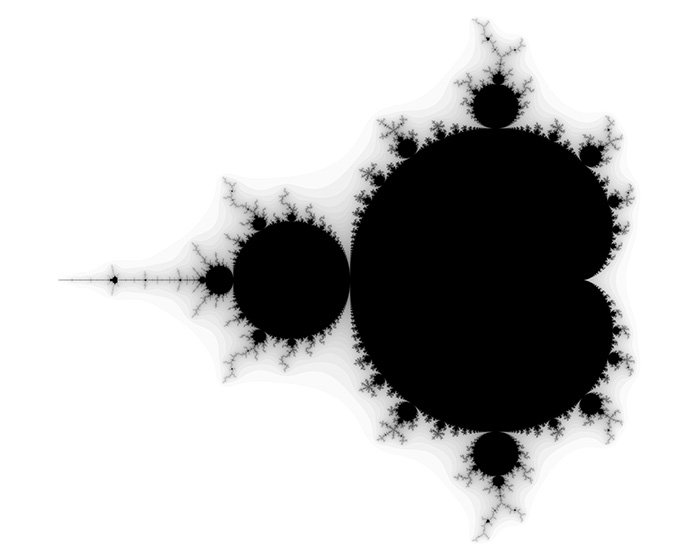zn+1 = zn2 + zo
The traditional Mandelbrot, M = 2.

M = 3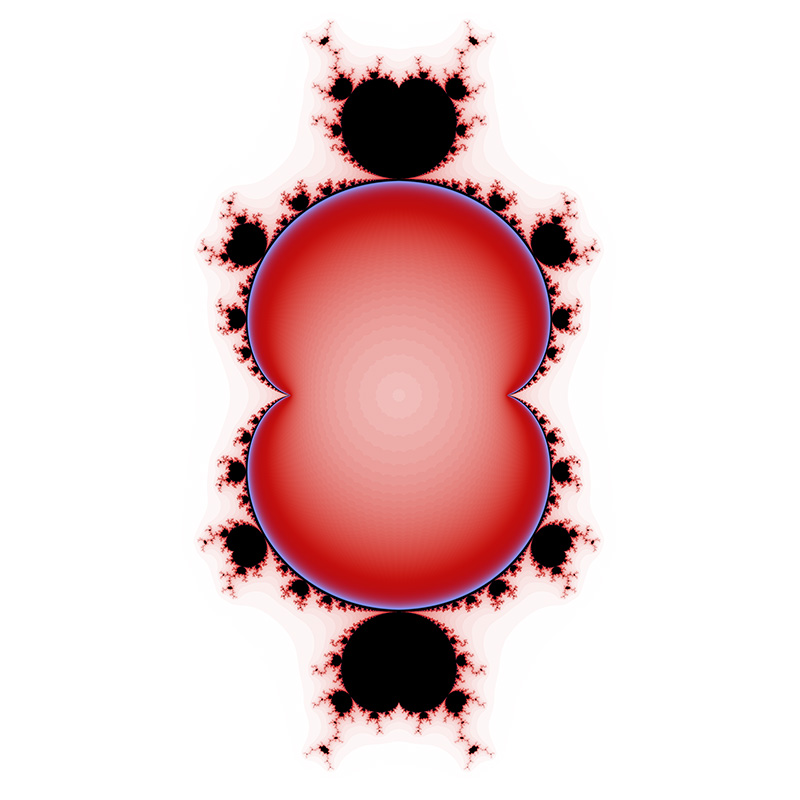zn+1 = zn3 + zo

M = 4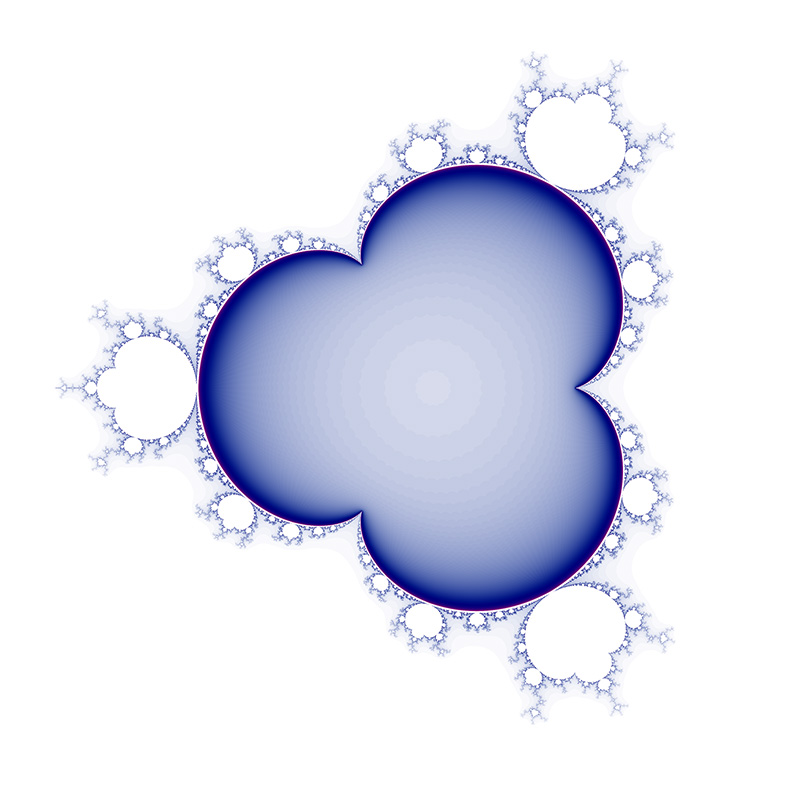zn+1 = zn4 + zo
In general there are M-1 main lobes which result in M-1 degrees of rotational symmetry.

M = 5zn+1 = zn5 + zo

M = 6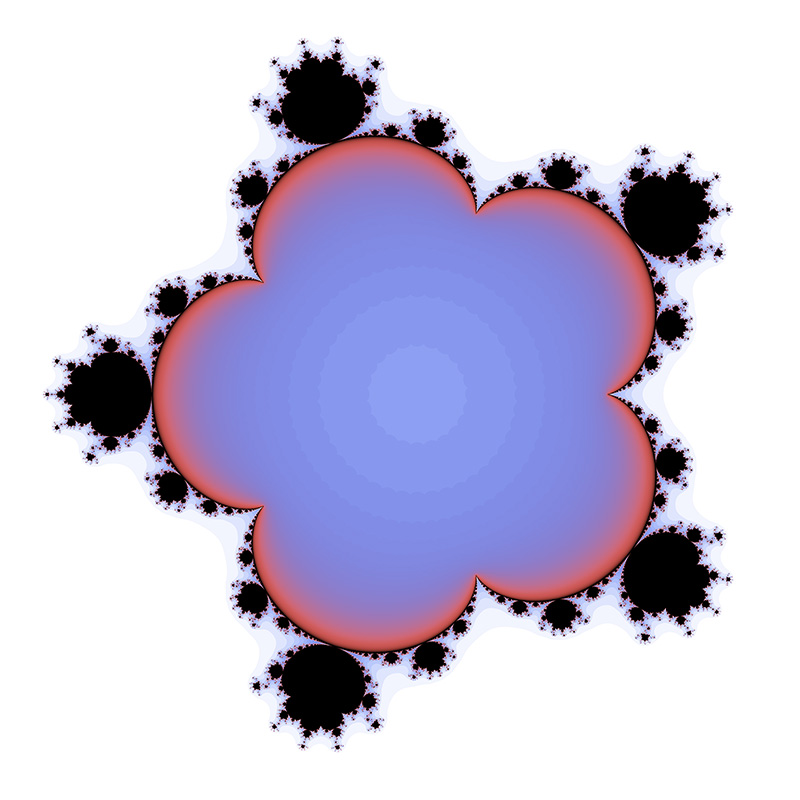zn+1 = zn6 + zo

M = 7zn+1 = zn7 + zo

M = 8zn+1 = zn8 + zo

M = 10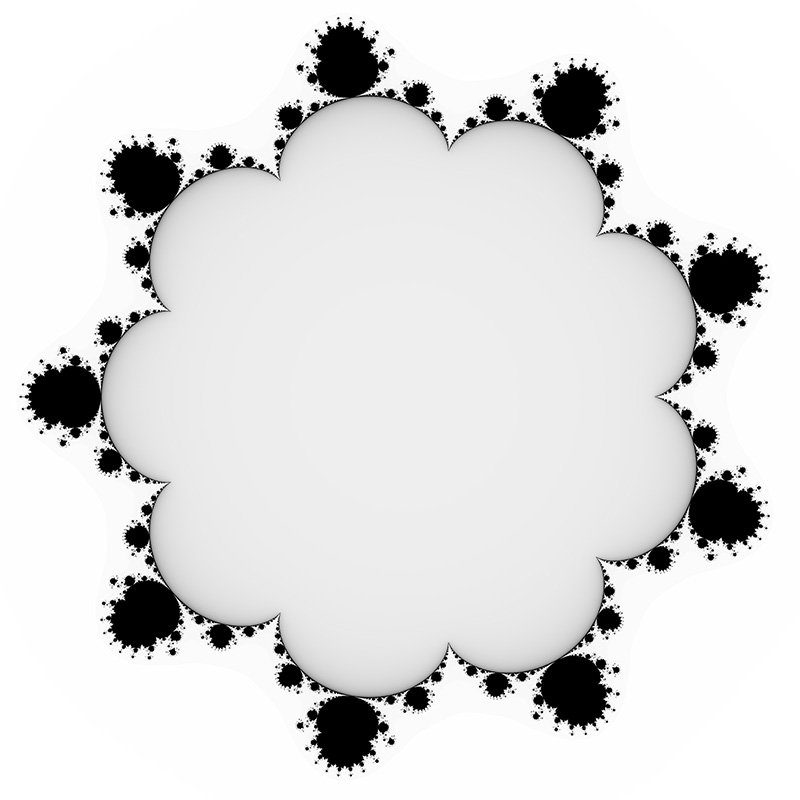zn+1 = zn10 + zo
The shapes get increasingly circular looking, but the detail on zooming in remains fractal.Non-Integer powers

M = 2.1zn+1 = zn2.1 + zo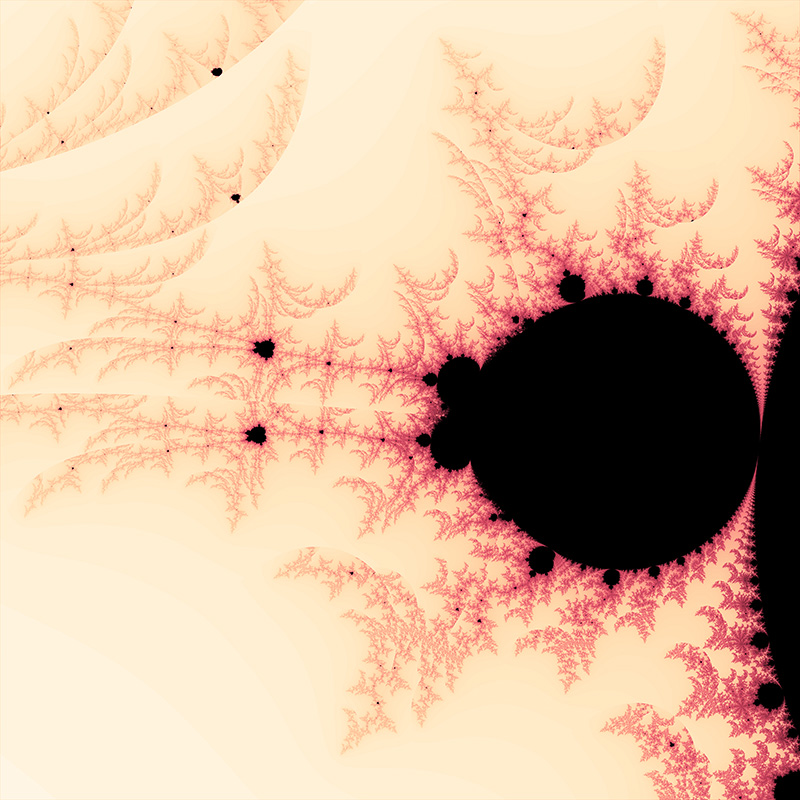M = 2.3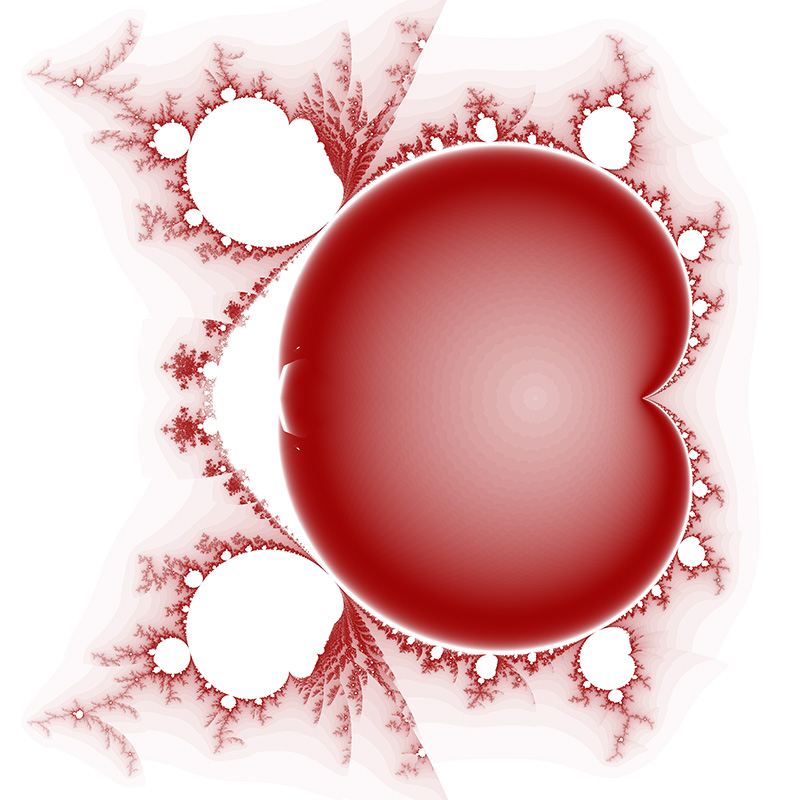zn+1 = zn2.3 + zo

M = 2.5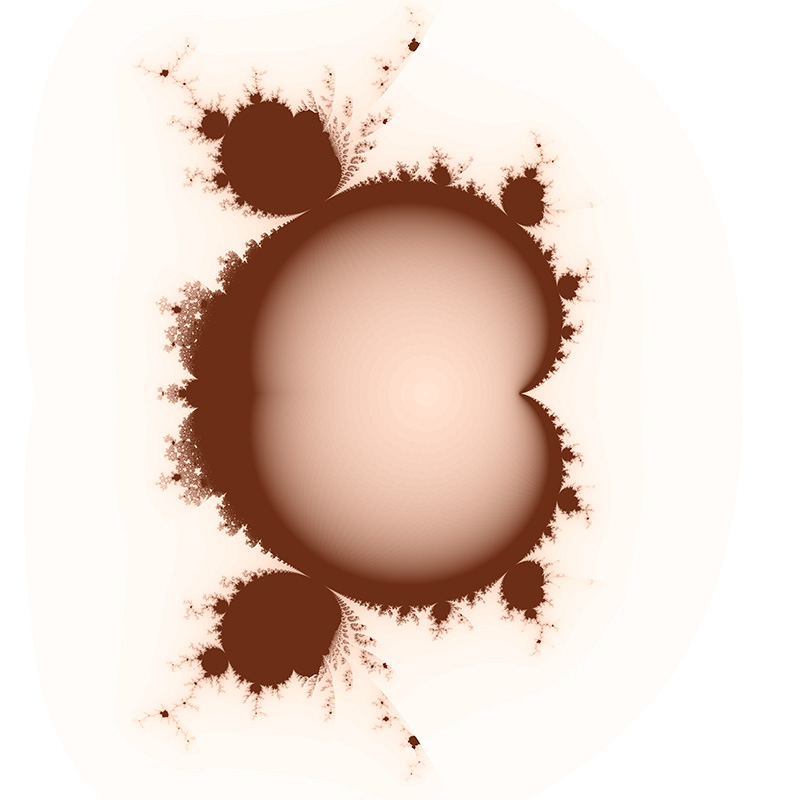zn+1 = zn2.5 + zo
Real valued M tend to appear like transitions between the lower and higher integer powers with a split along the negative real axis.

M = 2.7zn+1 = zn2.7 + zo

Negative powers

M = -1zn+1 = zn-1 + zo

M = -2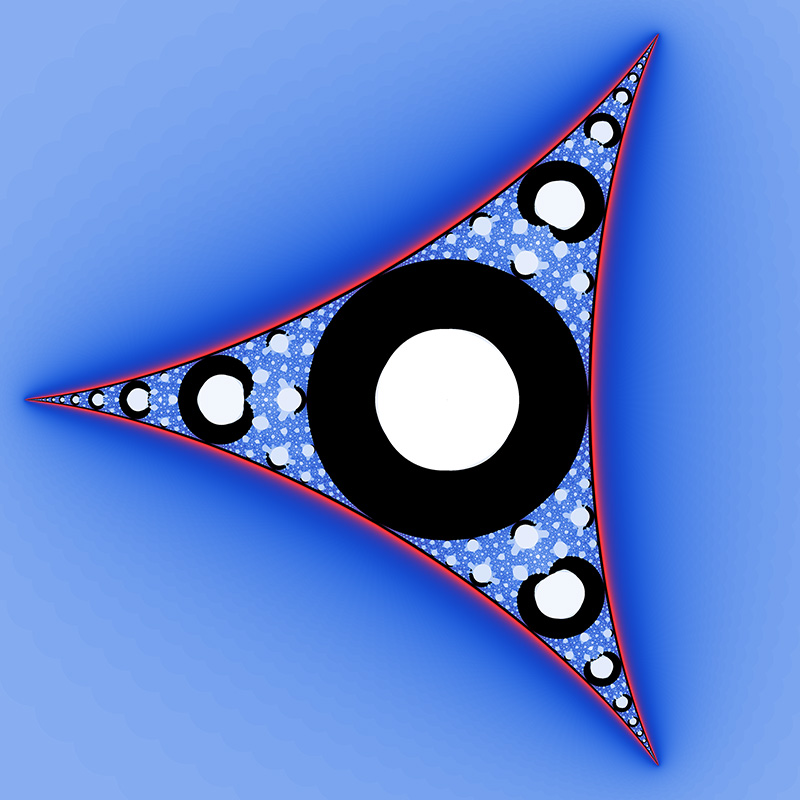zn+1 = zn-2 + zo

M = -3zn+1 = zn-3 + zo

M = -10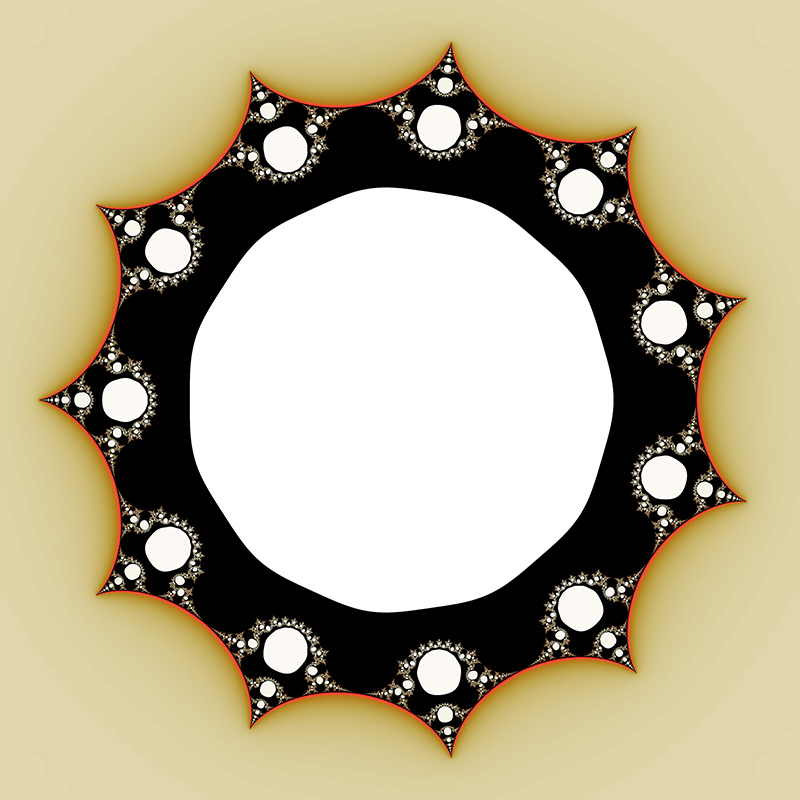zn+1 = zn-10 + zo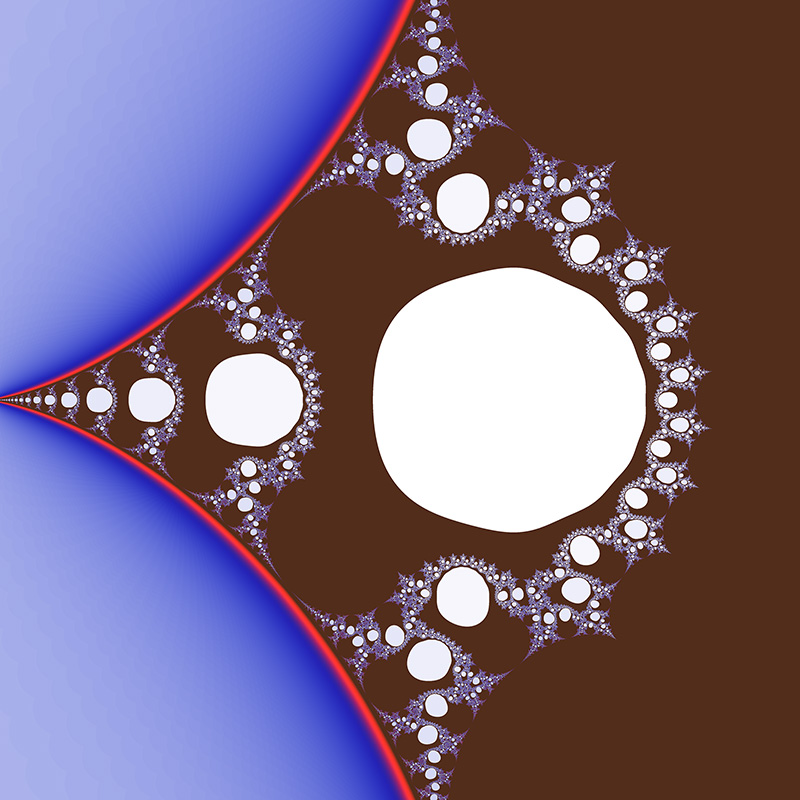M = -2.3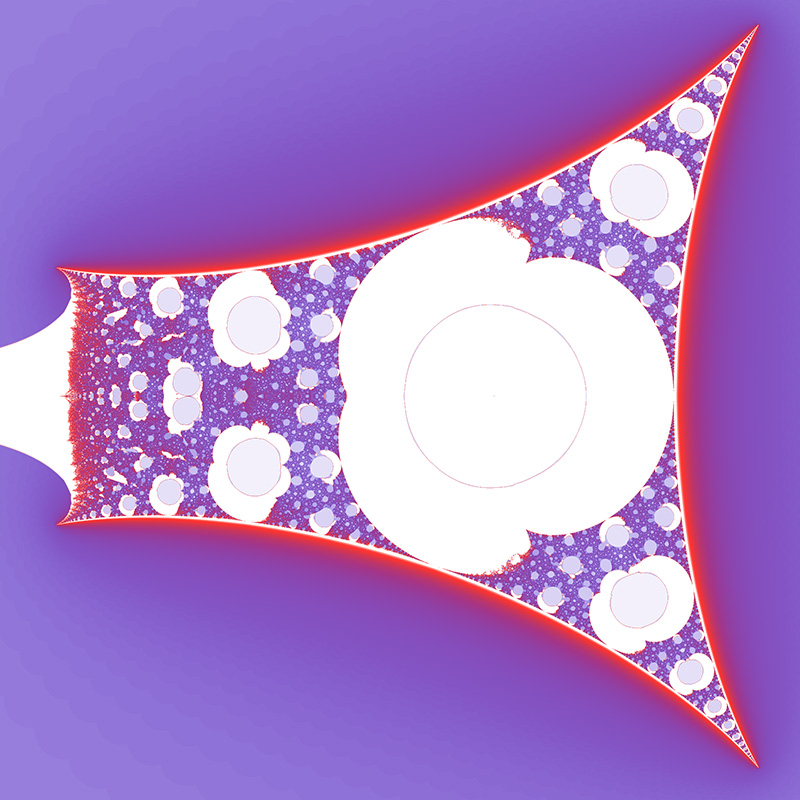zn+1 = zn-2.3 + zo

M = -2.5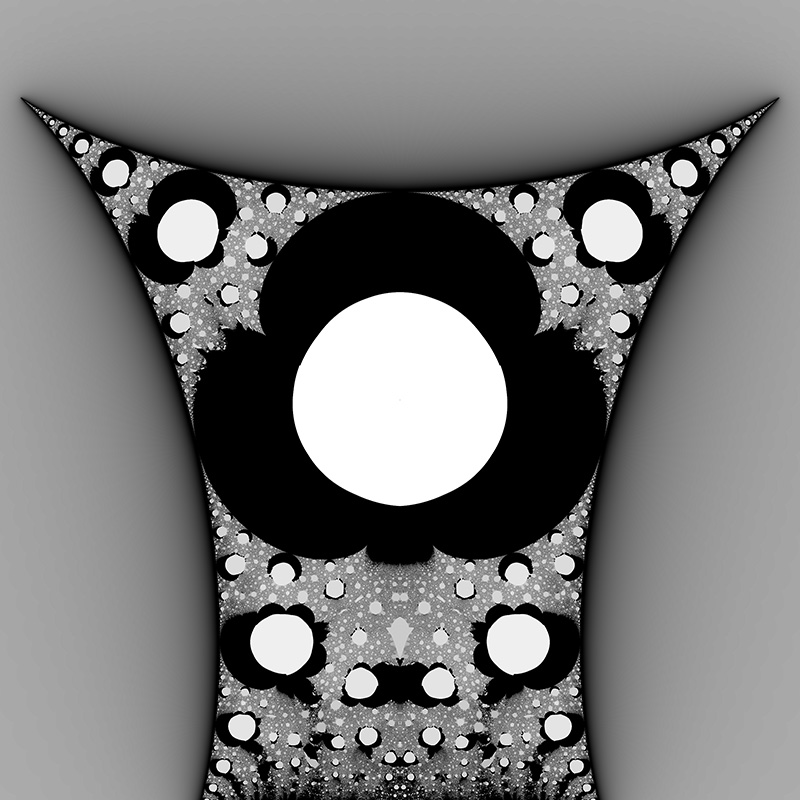zn+1 = zn-2.5 + zo